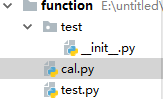• ## Python函数求导

万次阅读 多人点赞 2018-06-06 12:11:44
from sympy import *x ...) # 符号x，自变量y = -pow(10,-11)*pow(x,6) + pow(10,-8)*pow(x,5) - 4*pow(10,-6)*pow(x,4) + 0.0006*pow(x,3) - 0.0428*pow(x,2) + 1.7561*x + 16.528 #公式dify = diff(y,x) #求导pri...
from sympy import *x = symbols("x")  # 符号x，自变量y = -pow(10,-11)*pow(x,6) + pow(10,-8)*pow(x,5) - 4*pow(10,-6)*pow(x,4) + 0.0006*pow(x,3) - 0.0428*pow(x,2) + 1.7561*x + 16.528 #公式dify = diff(y,x) #求导print(dify)  #打印导数#给定x值，求对应导数的值for i in range(0,305,5):    print(dify).subs('x',i)
展开全文• python实现函数求导的方法是：1、利用sympy库中的symbols方法传入x和y变量；2、利用sympy库中的diff函数传入需要求导的函数即可返回求导之后的结果。python利用sympy库对某个函数求导，numpy库使用该求导结果计算的...
python实现函数求导的方法是：1、利用sympy库中的symbols方法传入x和y变量；2、利用sympy库中的diff函数传入需要求导的函数即可返回求导之后的结果。python利用sympy库对某个函数求导，numpy库使用该求导结果计算的程序在python数据处理过程中，我们经常会遇见这样一种情况。需要对一个函数表达式求偏导，并将具体数值代入导数式。而python中通常可用于函数求导的函数是sympy库中的diff()函数。但他通常所求得的导数只是一个符号表达式。不能直接带入数据使用。如下例：import sympy as spimport numpy as npx,y = sp.symbols('x y')z = sp.sin(2*sp.pi*x+2*y/5)zx = sp.diff(z,x)zy = sp.diff(z,y)print(zx)print(zy)其输出为：2*pi*cos(2*pi*x + 2*y/5)2*cos(2*pi*x + 2*y/5)/5那么该如何解决这个问题呢？对x,y使用evalf()函数分别赋值后，用float进行类型转换后，才能利用numpy进行数值计算。如下例：import sympy as spimport numpy as npx,y = sp.symbols('x y')z = sp.sin(2*sp.pi*x+2*y/5)zx = sp.diff(z,x)zy = sp.diff(z,y)x1 = 10y1 = 5z_x1 = float(zx.evalf(subs={x:x1,y:y1}))z_y1 = float(zy.evalf(subs={x:x1,y:y1}))print(z_x1)print(z_y1)其输出结果：-2.61472768902227-0.16645873461885696那如果我的x或y不是单一的值呢？而是一个数组。我们可以利用一个循环来完成。如下例：import sympy as spimport numpy as npx,y = sp.symbols('x y')z = sp.sin(2*sp.pi*x+2*y/5)zx = sp.diff(z,x)zy = sp.diff(z,y)x_array = np.linspace(-5, 5, 10)y_array = np.linspace(-5, 5, 10)temp_x = []#先定义一个用于存储x偏导的空列表temp_y = []#先定义一个用于存储y偏导的空列表for i in range(10):z_x = float(zx.evalf(subs={x:x_array[i],y:y_array[i]}))temp_x.append(z_x)#将计算得到的偏导值一一添加到列表中z_y = float(zy.evalf(subs={x:x_array[i],y:y_array[i]}))temp_y.append(z_y)zx_array = np.array(temp_x)#将列表转换为数组zy_array = np.array(temp_y)print(zx_array)print(zy_array)输出结果为：[-2.61472769  4.11163864  6.02946289  0.89585862 -5.2854481  -5.28544810.89585862  6.02946289  4.11163864 -2.61472769][-0.16645873  0.26175505  0.38384753  0.05703213 -0.33648208 -0.336482080.05703213  0.38384753  0.26175505 -0.16645873]由此便实现了由sympy得到求导结果，到numpy库进行数值计算。本人还是python初学者，有什么错误恳请各位大佬及时指正~学习路上共同进步~
展开全文• 通过给函数指定一个描述性的名称，并将函数存储在被称为模块的独立文件中，再将模块导入主程序中，通过import语句允许在当前运行的程序文件中使用模块中的代码，一个.py文件就称为一个模块，包含了Python对象定义和...
1.模块函数的优点之一，就是可以使用函数将代码块与主程序分离，通过给函数指定一个描述性的名称，并将函数存储在被称为模块的独立文件中，再将模块导入主程序中，通过import语句允许在当前运行的程序文件中使用模块中的代码，一个.py文件就称为一个模块，包含了Python对象定义和Python语句。如下就是一个简单的模块cal.pydef cal(x,y):return x+y导入模块的方法有很多种import module      #导入整个模块from module.xx.xx import xx     #导入模块中的特定函数from module.xx.xx import xx as rename    #使用as给函数指定别名from module.xx.xx import *    #导入模块中的所有函数(不推荐)对于上面一个模块，如果想要在另一文件中调用它，即:这种就通过import导入，但是调用的时候需要使用模块名.函数名的方式调用,即import calprint(cal.cal(3,5))那么函数再导入模块的时候是以哪个路径为基准呢？import sysprint(sys.path)通过sys.path输出的路径，就是寻找该模块的基准路径。模块分为三种- 自定义模块- 第三方模块- 内置模块- 自定义模块2.常用模块time时间有关的操作，有三种表达方式：1.时间戳(time.time)2.结构化时间(time.localtime)3.格式化的字符串(time.striftime)import timeprint(time.time())    #时间戳，是指从1970年1月1日0点0分到当前时间过了多少秒，常用来计算运行结果：1513730364.4014938print(time.localtime())   #结构化时间，通过这种方式，可以截取具体的年月日时分秒等信息运行结果：time.struct_time(tm_year=2017, tm_mon=12, tm_mday=20, tm_hour=8, tm_min=40, tm_sec=58, tm_wday=2, tm_yday=354, tm_isdst=0)t=time.localtime()print(t.tm_year)      #运行结果：2017 可以通过.关键字的方式，截取想要的信息print(time.strftime("%Y-%m-%d,%X"))    #格式化的字符串，必须写入想输出的时间格式运行结果：2017-12-20,08:48:01将元组形式的时间转化为字符串形式，返回时间形式为：Thu Dec 28 09:21:06 2017print(time.asctime())返回结果格式同asctimeprint(time.ctime())返回UTC时间结构化时间print(time.gmtime())三种表达方式的互相转化格式化的字符串转换成结构化时间：print(time.strptime("2017-12-20","%Y-%m-%d"))将结构化时间转换成时间戳print(time.mktime(time.localtime()))将结构化时间转换成格式化的字符串print(time.strftime("%Y-%m-%d %X",time.localtime()))random随机模块，主要功能如下:import random#随机生成一个0-1的浮点数print(random.random())#随机生成一个整数#生成一个指定范围内的随机浮点数print(random.uniform(10,20))#随机生成一个整数print(random.randint(1,9))#从指定范围内，按指定基数递增的集合中 获取一个随机数print(random.randrange(10,100,2))    #随机取10-100之间的偶数#从序列中随机获取一个元素print(random.choice(["tuple","list","dic"]))#将一个列表中的元素打乱p=["tuple","list","dic"]random.shuffle(p)print(p)随机数小案例 ，生成一个五位的随机数import randomdef random_code():res=""for i in range(5):num=random.randint(0,9)alf=chr(random.randint(60,100))result=str(random.choice([num,alf]))res+=resultreturn resprint(random_code())os模块提供对操作系统进行调用的接口，该模块的介绍我在linux操作系统下完成os.getcwd()                 获取当前所在路径os.chdir("test")            改变当前的工作路径，相当于cdos.makedirs('test/test1')   创建多级目录os.removedirs('test1')      递归删除，如目录为空，则删除os.mkdir('test')            创建单级目录os.rmdir('dirname')         删除单级空目录，目录不为空无法删除，报错os.listdir('dirname')       列出指定目录下的所有文件和子目录，包括隐藏文件，并以列表方式打印os.remove()                 删除一个文件os.rename("oldname","new")  重命名文件，用“，”分割os.stat('path/filename')    获取文件/目录信息os.sep                      操作系统的路径分隔符，win下为"\\",Linux下为"/"os.linesep                  当前平台使用的行终止符，win下为"\t\n",Linux下为"\n"os.pathsep                  用于分割文件路径的字符串os.name                     字符串指示当前使用平台。win->'nt'; Linux->'posix'os.system("bash command")   运行shell命令，直接显示os.environ                  获取系统环境变量os.path.abspath(path)       返回path规范化的绝对路径os.path.split(path)         将path分割成目录和文件名二元组返回os.path.dirname(path)       返回path的目录。其实就是os.path.split(path)的第一个元素os.path.basename(path)      返回path最后的文件名。如何path以／或\结尾，那么就会返回空值。即os.path.split(path)的第二个元素os.path.join(path1[, path2[, ...]])  将多个路径组合后返回，第一个绝对路径之前的参数将被忽略sys模块sys.argv           命令行参数List，第一个元素是程序本身路径sys.exit(n)        退出程序，正常退出时exit(0)sys.version        获取Python解释程序的版本信息sys.maxint         最大的Int值sys.path           返回模块的搜索路径，初始化时使用PYTHONPATH环境变量的值sys.platform       返回操作系统平台名称sys.stdin          输入sys.stdout         输出sys.stderror       错误进度百分比小案例import sysimport timedef calc(num, total):rate = float(num) / float(total)     #float 浮点数关键字rate_num = int(rate * 100)r = '\r%d%%' % (rate_num, )          #要加上/r转义符，表示将输出的内容返回到第一个指针，即覆盖前面的内容sys.stdout.write(r)sys.stdout.flush()if __name__ == '__main__':              #这条语句，如果用在被调用文件里面，用于被调用文件的一个测试for i in range(0, 101):                #  如果放在执行文件里面，就是不想让该文件让齐塔程序调用time.sleep(0.1)calc(i, 100)进度条小案例import sysimport timefor i in range(100):sys.stdout.write("#")time.sleep(0.1)sys.stdout.flush()因为工作原因，最近一周没有更新博客，我会在这周补回来，下一篇文章我会重点写re模块。
展开全文• 使用python编写的py文件b.已被编译为共享库或DLL的C或C++扩展c.把一系列模块组织到一起的文件夹(注：文件夹下有一个__init__.py文件)d.使用C编写并链接到python解释器的内置模块模块分为三大类：a.自定义模块b.内置...
一、模块的使用1.什么是模块模块就是一系列功能的集合体模块的表现形式为：a.使用python编写的py文件b.已被编译为共享库或DLL的C或C++扩展c.把一系列模块组织到一起的文件夹(注：文件夹下有一个__init__.py文件)d.使用C编写并链接到python解释器的内置模块模块分为三大类：a.自定义模块b.内置模块c.第三方模块2.为什么要用模块a.可以下载别人的模块，然后直接使用。拿来主义。会极大的提高开发效率b.将程序公用的功能组织到一个文件中，便于使用和管理3.怎么用一个py文件就是一个模块，若文件名为spam.py，则模块名为spamimport 导入首次import导入模块会发生三件事：a.创建一个模块spam.py的名称空间b.执行模块对应的文件spam.py，将产生的名字丢到模块的名称空间中c.在当前执行文件的名称空间中拿到一个名字spam,该名字就是指向模块spam.py的名称空间。在当前执行文件中引用模块中的名字：模块名 如：spam.名字ps：a.重复导入无效b.函数作用域关系在定义阶段就固定死了，与调用阶段无关\from .... import :为什么要这个？ 简化import代码量首次from...import导入模块会发生三件事：a.创建一个模块spam.py的名称空间b.执行模块对应的文件spam.py，将产生的名字丢到模块的名称空间中c.将变量与spam名称空间的 变量地址绑定注意：1.run.py的同变量名会在当前名称空间查找。2.函数的作用域在定义阶段就固定死了两种导入方式的对比：相同点：a.函数的作用域关系在定义阶段就固定死了，与调用位置无关b.都创建对应的模块名称空间，都会执行一次py文件、初始化名称空间不同点：from...import：优点：from...import 可以不用加前缀而直接引用名字，更简洁缺点：容易与当前执行文件的名字冲突import:优点：指名道姓，肯定不会与当前执行文件的名字冲突缺点：必须加前缀as 关键字改名：from ... import *:* 会检索被导入模块中的__all__指定的名字，如果没指定，默认导入所有变量二、循环导入的问题模块循环/嵌套导入抛出异常的根本原因是由于在python中模块被导入一次之后，就不会重新导入，只会在第一次导入时执行模块内代码在我们的项目中应该尽量避免出现循环/嵌套导入，如果出现多个模块都需要共享的数据，可以将共享的数据集中存放到某一个地方在程序出现了循环/嵌套导入后的异常分析、解决方法如下示例：m1.py文件m2.py文件run.py执行执行得到结果:出现原因:先执行run.py--->执行import m1，开始导入m1并运行其内部代码--->打印内容"正在导入m1"--->执行from m2 import y 开始导入m2并运行其内部代码--->打印内容“正在导入m2”--->执行from m1 import x,由于m1已经被导入过了，所以不会重新导入，所以直接去m1中拿x，然而x此时并没有存在于m1中，所以报错解决方法1：导入语句放到最后，把所有名字的定义放到前面解决方法2：导入语句放到函数中三、区分python文件的两种用途__name__：python内置变量。在满足某种条件自动触发使用。1.可以直接执行运行当做执行文件执行了：__name__ == '__main__'2.可以被当做模块导入:当做模块被导入时：__name__ == '模块名'：四、模块的搜索路径1.优先从内存中查找:a.导入模块spamb.sleep 20秒c.重新执行代码2.其次查找内置模块3.再次去 sys.path的环境变量里面依次查找ps：导入模块一定要搞清楚执行文件是谁和被导入模块是谁。sys.path第一个元素是以当前执行文件的路径为准a.导入文件可以向sys.path append或inert路径b.以执行文件路径为准，from...import模块展开全文• oo>>> limit(1/sin(x), x, oo) #极限不存在AccumBounds(-oo, oo) 求导>>> diff(cos(x), x)-sin(x)>>> diff(cos(x)*sin(y), x, x, x) #多次求导(对x连续求3次偏导数)sin(x)*sin(y)>>> diff(cos(x)*sin(y), x,3)...
• python实现函数求导的方法是：1、利用sympy库中的symbols方法传入x和y变量；2、利用sympy库中的diff函数传入需要求导的函数即可返回求导之后的结果。python利用sympy库对某个函数求导，numpy库使用该求导结果计算的...
• python实现函数求导的方法是：1、利用sympy库中的symbols方法传入x和y变量；2、利用sympy库中的diff函数传入需要求导的函数即可返回求导之后的结果。python利用sympy库对某个函数求导，numpy库使用该求导结果计算的...
• python实现函数求导的方法是：1、利用sympy库中的symbols方法传入x和y变量；2、利用sympy库中的diff函数传入需要求导的函数即可返回求导之后的结果。python利用sympy库对某个函数求导，numpy库使用该求导结果计算的...
• 前文提到了神经网络中的Sigmoid函数，实际上在反向传播中还会用到Sigmoid的导数，形式很简单: s(x)*(1-s(x))，但是我想把这个过程自己推导一次，顺便复习一下导数和微分。Derivative(导数)和Differential(微分)首先...
• python numpy 多项式函数 求导求根 """ 求出多项式的 导函数与根 """ import numpy as np import matplotlib.pyplot as mp # 多项式函数 y = 4x^3 + 3X^2 - 1000x + 1 P = [4, 3, -1000, 1] # 多项式系数 数组 x ...
• Let us consider the following function:$f(x)=\begin{cases} 0,& \Pr(f(x)=0)=x \\1,& \Pr(f(x)=1)=1-x\end{cases}$,where $0< x< 1$Trial:I have tried with the following code but I,m not sur.....
• 由于闭包和 Python 在对象自省上的灵活性，我们可以提炼出 operator 函数的创建。 # 一个创建闭包的函数，实现了二元运算的逻辑 _MISSING = object() def _create_binary_op(name: str, operator: str) -> Any: ""...
• interval -interval n= float (2 ** self.chromosome_size -1)return (interval + chromosome * d /n)deffitness_func(self, chrom1, chrom2):'''适应度函数，可以根据个体的两个染色体计算出该个体的适应...
• 利用python函数求导数的方法：首先python运行环境，导入微分的模块包；然后定义符号变量和函数；接着求导【diff = diff(f,x)】；**后输入diff就显示其变量值了。fuG少儿编程网-https://www.pxcodes.comfuG少儿编程网...
• 利用Python对自定义函数求导一、题目描述二、解题步骤运行环境题目分析三、完整代码四、运行结果 一、题目描述 自定义一个函数，对函数求一阶和二阶导数，并绘制原函数和求导函数的函数图像 二、解题步骤 运行环境 ...
• #高阶函数#变量可以指向函数#以Python内置的求绝对值的函数abs()为例，调用该函数用以下代码print(abs(-10))#只写abs#输出为：print(abs)#abs(-10)是函数调用，而abs是函数本身#要获得函数调用结果，我们可以把结果...
• 函数本身也可以赋值给变量，即：变量可以指向函数。Eg:>>> f = abs>>> f如果一个变量指向了一个函数，那么，可通过该变量来调用这个函数。Eg:>>> f = abs>>> f(-10)10函数名也...
• 我正在尝试重新实现我在论文中阅读的方法，但我不确定如何做一些方面 . 它基于最小二乘多项式拟合及其导数 . 我有我的输入数据，以便： x = [ 421.25571634 426.... 我理解差异化但不确定如何在python中做到这一点 .
• Python——函数式编程、高阶函数及内置函数函数式编程一、不可变数据：不用变量保存状态不修改变量二、第一类对象：函数即“变量”1、函数名可以当做参数传递2、返回值可以是函数名三、尾调用优化(尾递归)： 在函数...
• 变量可以指向函数Python内置的求绝对值的函数abs()为例，调用该函数用以下代码：>>> abs(-10)10但是，如果只写abs呢？>>> abs可见，abs(-10)是函数调用，而abs是函数本身。要获得函数调用结果，我们可以把结果赋值...
• 高阶函数函数作为参数传入，这样的函数称为高阶函数函数式编程就是指这种高度抽象的编程范式。变量可以指向函数函数的参数能接收变量，那么一个函数就可以接收另一个函数作为参数，这种函数就称之为高阶函数。...
• 梯度下降法和牛顿法的总结与比较机器学习的本质是建立优化模型，通过优化方法，不断迭代参数向量，找到使目标函数最优的参数向量。最终建立模型通常用到的优化方法：梯度下降方法、牛顿法、拟牛顿法等。这些优化方法...
• 更多python教程请到： 菜鸟教程www.piaodoo.com人人影视www.sfkyty.com16影视www.591319.com星辰影院www.591319.compytorch中自定义backward()函数。在图像处理过程中，我们有时候会使用自己定义的算法处理图像，...
• 我试过很多拟合函数，包括多项式拟合和一元样条曲线拟合，但这两种方法都没有使我产生我想要的拟合。在所以，我在找一个曲线拟合函数(在scipy中？)这将允许我定义最终曲线的已知约束。下面是我的数据示例，其中有一......

# python函数求导python 订阅